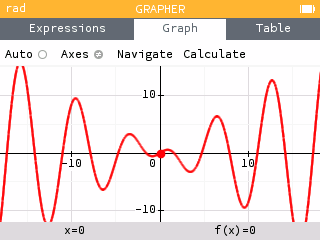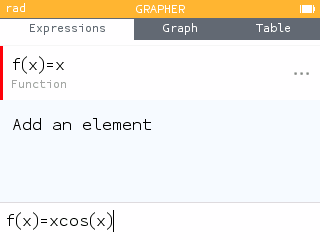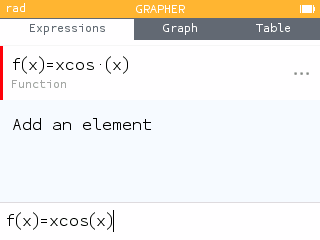# An Improved Parser!

At some point in your mathematical journey, you may be interested in graphing the function `f(x)=xcos(x)`. It’s a pretty neat looking graph!However, before the release of software version 19, you may have ran into some issues. A blank graph and a table full of “undef” may have left you wondering what went wrong!To understand this issue, and its fix, we must first consider what the calculator is doing whenever you press exe.

## Understanding the parser

In her post To develop is to renounce!, Léa gave us a great insider’s view of what happens when you press exe on the NumWorks calculator.

One of the first things the calculator does is try to understand what you have written. If you write `13+2`, the calculator first identifies the different ‘words’ in the calculation, which are the number `13`, the symbol `+`, and the number `2`. It then applies some grammar rules. You are doing this now by reading and comprehending this sentence. A number, followed by `+`, followed by a number, is the addition of the first number with the second.

This strategy of breaking down a statement into parts to understand it is called parsing.

Léa showed us that when we introduce variables and functions, things get complicated.
She has us consider `abba(1+2)`:

• Is it the function `abba` applied to `1+2`?
• Is the variable `abba` multiplied by `1+2`?
• Is it the variable `a` multiplied by the variable `b`, multiplied by the variable `b`, multiplied by the variable `a`, multiplied by `1+2`?
• Is it the variable `abb` multiplied by the function `a` applied to `1+2`?

Prior to version 19, our parser followed the rule: Always create the longest variable name possible and, if it is followed by parentheses, it is a function name. This rule is simple, but the user will have to write multiplication signs where they could be implicit.

And therein lies the issue you may have found when graphing `f(x)=xcos(x)`.Here, without the explicit multiplication between `x` and `cos(x)`, the parser looks for the longest variable name, `xcos`. However, since `xcos` is not defined, we end up with the unknown variable `xcos` multiplied by the variable `x`, that is, `xcos*(x)`. Since `xcos` is undefined, the function itself is undefined.

## A proposed solution

When problem solving, it is important to consider several solution methods. When first approaching this problem, we considered automatically preceding functions such as `sin()`, `cos()`, `ln()`, etc with a multiplication symbol when using the keys or toolbox (e.g. `*sin()`). However, this would not solve the problem when these functions are typed in manually using the alpha keys or a computer keyboard on the emulator.

## A better fix: Improve the parser!

To create a more universal fix, we needed to make adjustments at the parser level. This involved redesigning our parsing algorthim.

### Implicit multiplication by default

By default we consider that `ab` means `a*b`. That is, unless `ab` is a defined variable or function, we assume implicit multiplicaton. (More on that below!)

To avoid a regression in the Solver application, we now support variable strings using quotation marks: `"apple"+"pear"=12`.

### Right-to-left eager tokenizer

When parsing a string `abc` we’ll first consider `abc`, then `bc`, then `c` until we reach a defined function or variable or arrive at a single character. We then repeat this algorithm with the remaining string.

Consider our original function `f(x) = xcos(x)`. How does our new parser read `xcos`?Let’s consider some more examples.

The following symbols are defined: `a`, `b`, `ab`, `azfoo` and `foobar`. Also `cos()` and `acos()` are predefined functions.

1. How does our new parser read `abfoobar(x)`?While `a` and `b` are also defined, the parser will consider the entire string `ab` first. If you want to multiply `a` by `b`, you will need to use the explicit multiplication symbol: `a*b`

2. How does our new parser read `acos(x)`?Here `a` and `cos` are also defined. However, the parser will consider the entire string `acos` first which is a predefined function. If you want to multiply `a` by `cos`, you will need to use the explicit multiplication symbol: `a*cos`

3. How does our new parser read `azfoobar(x)`?In this last example, you may wonder why the defined symbol `azfoo` was not used. The parsing algorithm will eliminate the left most characters first. We could have gone in the other direction, but this behavior is a convenient way to give precedence to function over symbols. This gives us `a*z*foobar(x)` instead of `azfoo×b×a×r×(x)`.

Explore the calculator yourself! Look out for how the parser evaluates your input and do not hesitate to suggest improvements!##### Nick Koberstein — Math Teacher in Residence

Nick is one of our Math Teachers in Residence for the United States. He taught high school mathematics for nine years in North Carolina including Algebra 1 through AP Calculus and AP Statistics. Nick creates classroom resources for teachers, leads workshops and webinars, and collaborates with the engineering team to ensure our calculator meets the needs of teachers and students. In addition to educational technology, Nick’s other passions include music, board games, and growing avocado trees!